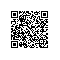# 求证：a^4+b^4 ≧a^3*b+a*b^3

a4+b4-a3b-ab3

=a3(a-b)-b3(a-b)

=(a3-b3)(a-b)

=(a-b)2(a2+ab+b2)使用钉钉扫一扫加入圈子
+ 订阅